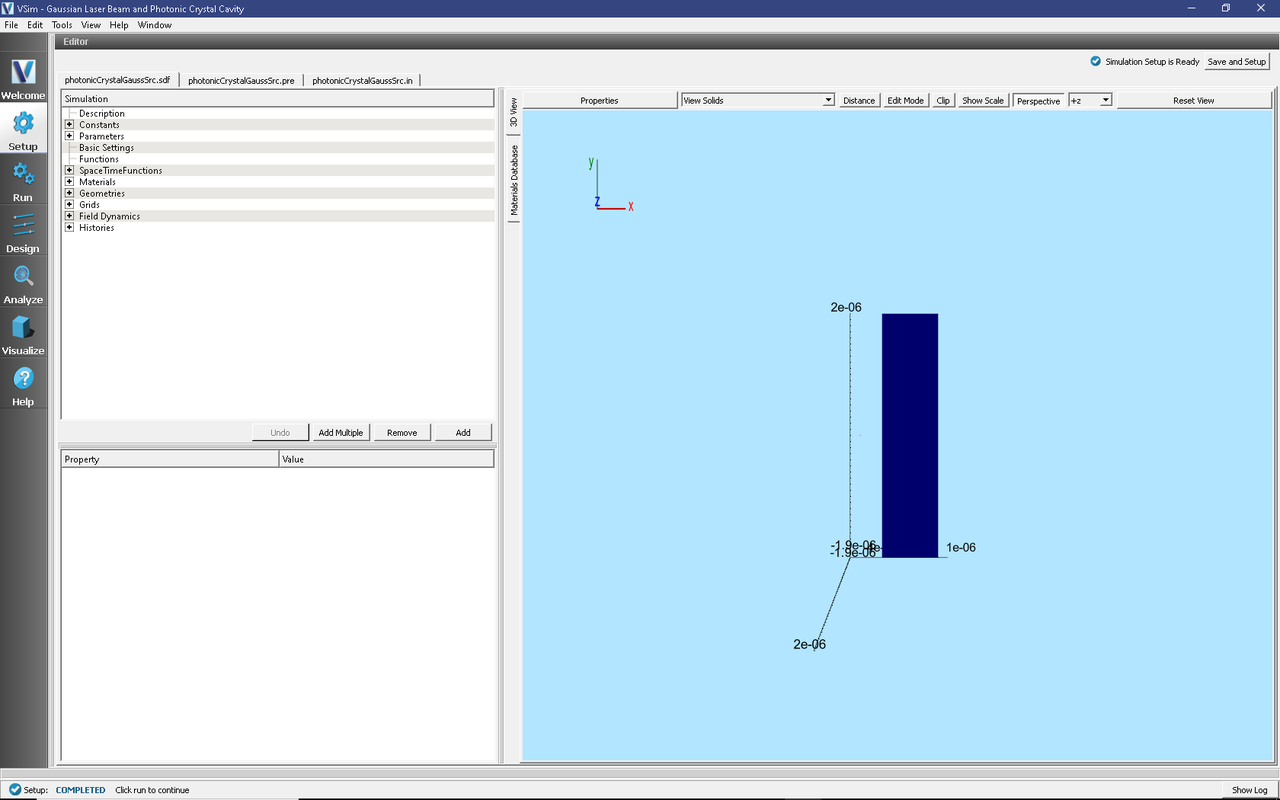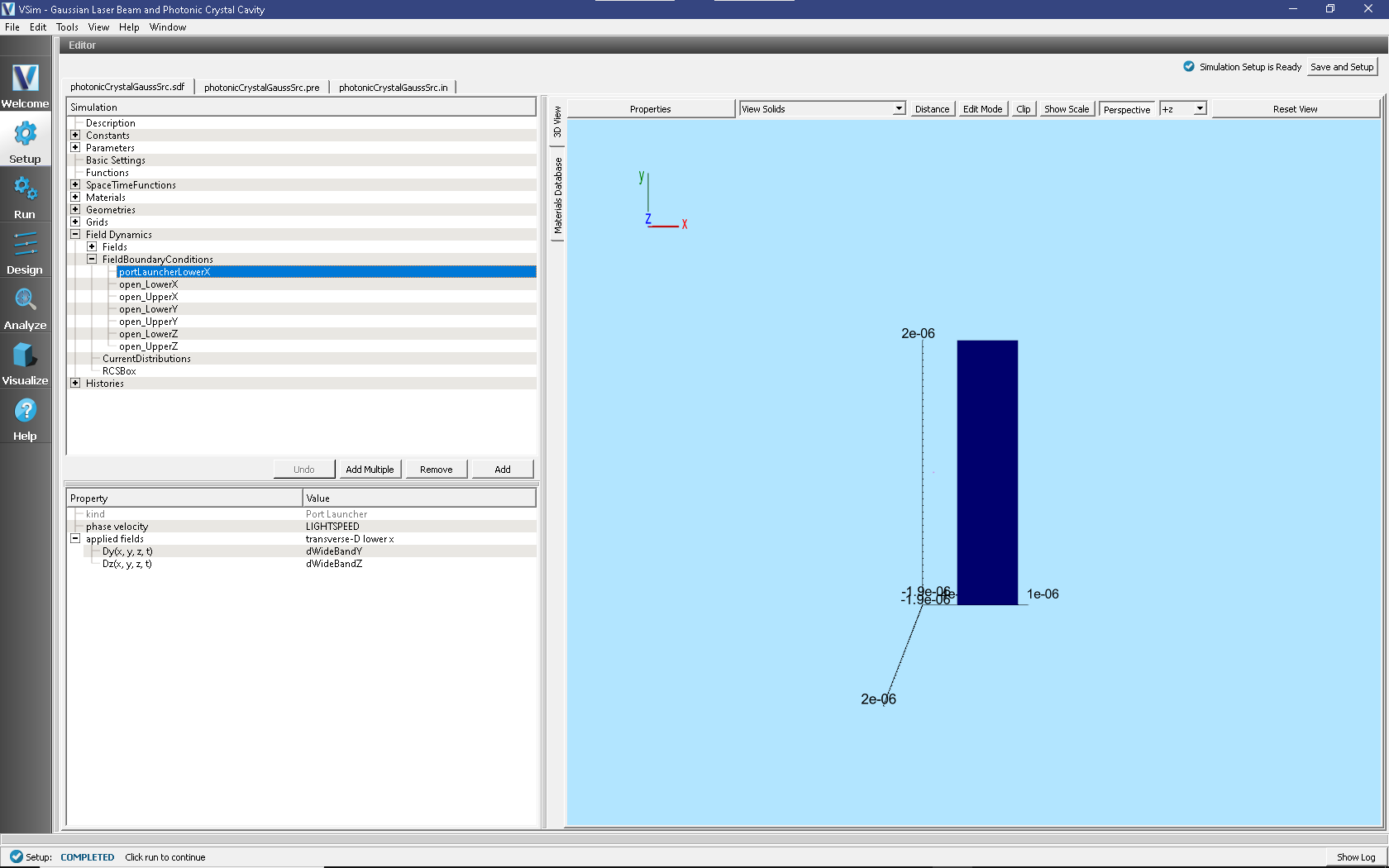# Gaussian Laser Beam and Photonic Crystal Cavity (photonicCrystalGaussSrc.sdf)

Keywords:

Gaussian Beam source, photonic crystal, transmission efficiency

## Problem description

This example illustrates how to model a Gaussian beam source that is illuminating a cavity inside a hexagonal photonic crystal lattice. The physical setup is shown in Fig. 254.

A Gaussian beam is launched from above into the simulation domain, which comprises three layers: a vacuum region above and a solid dielectric below, which together sandwich a central dielectric layer that contains a lattice of holes. This example includes two possible time signals with which the Gaussian beam will have either WideBand or SingleFrequency.

This simulation can be performed with a VSimEM license.

## Opening the Simulation

The Photonic Crystal example is accessed from within VSimComposer through the following steps:

• In the resulting Examples window, expand the VSim for Electromagnetics option.
• Expand the Photonics option.
• Select “Gaussian Laser Beam and Photonic Crystal Cavity” and press the Choose button.
• In the resulting dialog, create a new folder if desired, and press the Save button to create a copy of this example.

All of the properties and values that create the simulation are now available in the Setup Window, as shown in Fig. 255. You can expand the tree elements and navigate through the various properties, making any changes you desire. The right pane shows a 3D view of the geometry, if any, as well as the grid, if actively shown. To show or hide the grid, expand the Grid element and select or deselect the box next to Grid.Fig. 255 The Setup Window for the Gaussian Laser Beam and Photonic Crystal Cavity example

## Simulation Properties

This example contains a number of constants that are defined to make the simulation easily modifiable, as can be seen in Fig. 256.

All the following constants should be the only properties you should need to alter in order to specify your simulation domain.

General Simulation Parameters:
• L{X,Y,Z} = The length of your simulation domain in the {X,Y,Z} dimension.
• HT_{VACUUM,SI,SI02} = The height of the vacuum, SI and SI02 layers of the photonic crystal.
Source Specifications: (located in the Parameters section of the Elements Tree)
• {K_THETA,K_PHI,E_CHI} = The {polar angle, azimuthal, angle of polarization} respectively.
• WAVEL_CENTER = The central wavelength of your wideband signal. This is also the frequency used in the single frequency simulation type.
• WAVEL_BAND = The wavelength width of your wideband signal, only used in wideBand simType.
• BEAM_WAIST = The width at which your beam power falls off like 1/e.
• BEAM_POWER = The amplitude of your E/M wave.
• GAUSS_ORIGIN_{X,Y,Z} = The point around which your Gaussian profile is centered.
• TURNONCYCLES = The number of cycles you want your single frequency to reach full power.
• SIMCYCLES = Number of wave cycles you want your simulation to run.
• CYCLESPERDUMP = Number of cycles between each dump in the simulation.

The tool used to input the wave into the simulation is a port launcher. It specifies the Electric Displacement Field (D) at a boundary in this case the lower X boundary. The functions defining your D on the boundary are defined in the SpaceTimeFunctions element of the Elements Tree.

SpaceTimeFunctions:
• dSingleFreq{Y,Z} = This is the {x,y,z} component of the single frequency Gaussian beam source, as seen in Fig. 256. You put this as a parameter in the current distribution.
• dWideBand{Y,Z} = This is the {x,y,z} component of the wideband Gaussian beam source, as seen in Fig. 256. You put this as a parameter in the current distribution.

To choose which signal you want to input into this example simulation:

1. Expand the FieldBoundaryConditions element.
2. Left click on the portLauncherLowerX condition.

At this point, you should see what is shown in Fig. 257.Fig. 257 The Setup Window for specifying the signal for the portLauncher

1. To add a signal to the portLauncherLowerX just right click on the D{x,y} and select Assign SpaceTimeFunction. This will expand another menu that will show you all four defined SpaceTimeFunctions. Select which one you want to input into your simulation. For this documentation, WideBand{Y,Z} will be used to demonstrate the functionality of this example.

## Running the Simulation

After performing the above actions, continue as follows:

• Proceed to the Run Window by pressing the Run button in the left column of buttons.

• One can enable MPI options to utilize multi-core systems.

• The default values of Number of Time Steps and Dump Periodicity are taken from the parameters STEPSTOTAL and STEPSPERDUMP, which use the constants SIMCYCLES and CYCLESPERDUMP. The formulae for these variables can be found back in the Setup Window. These variables are for convenience to calculate good default values and it is important to know that the override option default values ultimately come directly from the numbers in the Basic Settings section.

Number of Steps and Dump Periodicity. Just copy these values into the correct fields in the Run menu.

• Number of Steps = TOTALSTEPS = 32220
• Dump Periodicity = STEPSPERDUMP = 3600
• To run the file, click on the Run button in the upper left corner of the Logs and Output Files pane. You will see the output of the run in the right pane. The run has completed when you see the output, “Engine completed successfully.” This is shown in Fig. 258.Fig. 258 The Run Window for the Gaussian Laser Beam and Photonic Crystal Cavity example

## Visualizing the results

After performing the above actions, continue as follows:

• Proceed to the Visualize Window by pressing the Visualize button in the left column of buttons.

In the simulation, there are specific grid points which store field histories. These histories are placed in various positions of the simulation.

• Click the Add a Data View dropdown below the menu bar and select History.

In Fig. 259, one can see there are 4 possible graphs to view at one time in the Visualize Window. For each graph, one can select the following fields to analyze: (0 = x, 1 = y, 2 = z).

• {E,B}_AtDet_{0,1,2} is in the middle of (y,z) plane and 60 nm above the surface of the crystal.
• {E,B}_AtSrc_{0,1,2} is aligned with the inCav history in the (y,z) plane and is 60 nm below the Si layer, into the SiO2 layer.
• {E,B}_InCav_{0,1,2} is slightly offset from the middle of one of the cavities in the silicon layer (the layer with the lattice).

In each individual graph, one can choose the Fourier Amplitudes (dB) option to view the frequency domain of your field. This can enable the analysis of the frequency response of the photonic crystal cavity.

Fig. 259 depicts four graphs of histories. The first two graphs are amplitude vs time, and the second two are Fourier Amplitudes of the first two on a log scale.

The first and third graphs depict the history AtSrc_2, while the second and fourth graphs show the AtDet_2 history.Fig. 259 The Visualize Window for the Gaussian Laser Beam and Photonic Crystal Cavity example

## Further Experiments

By using the wideband source and examining the field strength detected below the crystal lattice, one may study the frequency response of this photonic crystal as one changes the device geometry, the dielectric constants, and the location and polarizations of the radiation source and detector.